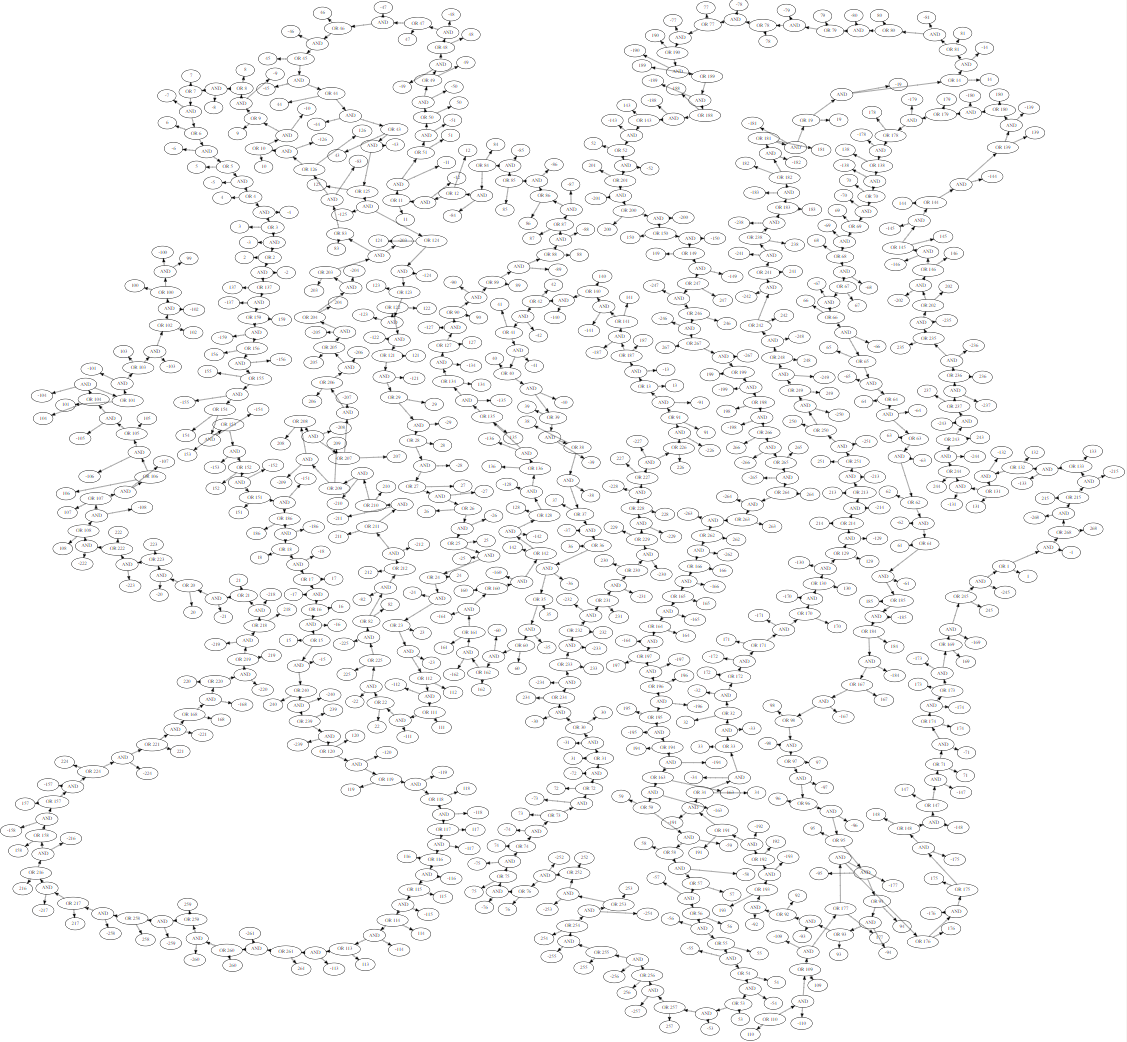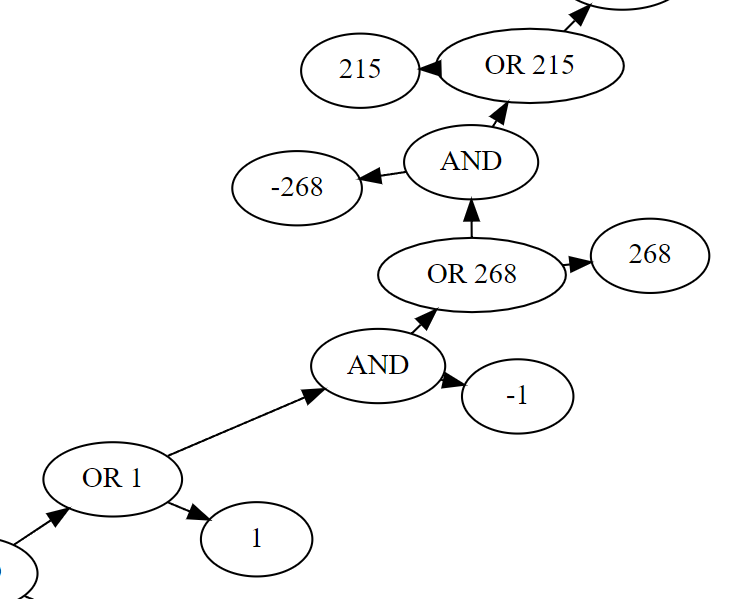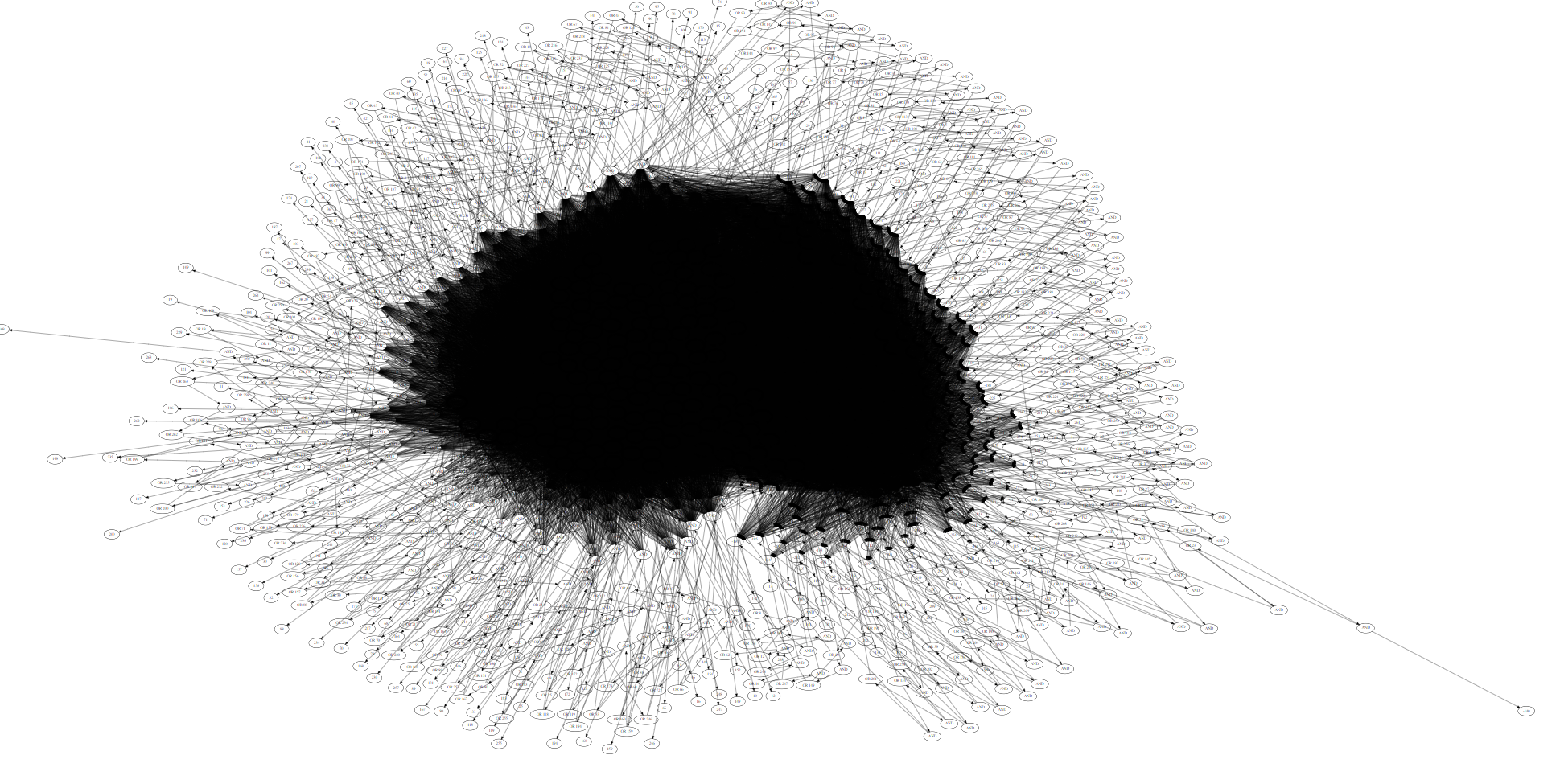# Agent Behaviour Modelling

### Eileen Yang

This post contains a re-implementation of ideas from the following paper: Modeling Blackbox Agent Behaviour via Knowledge Compilation using the Bauhaus library. The overarching idea was to capture the agent’s behaviour by taking trace data, a sequence of bit-vectors representing the state of the world (from the MACq (Model Acquisition) library) and pairing each state with actions taken to transition between said states. The original implementation code from the paper was unavailable, calling for a re-implementation for reproducibility.

## Summary

The paper contributes to explainable AI and builds an understanding of how agents behave through capturing the policy an agent is following. The ‘black box’ agent in this setting could range in complexity, anywhere from a human agent to a neural network - the paper makes no assumptions on the agent’s actual implementation of said policy. The approach represents the mapping of states to actions using a modern knowledge compilation technique: d-DNNF (disjunctive decomposable negation normal form), with the subsequent goal to answer inferential questions about the underlying logic of the agent’s decisions.

### Why d-DNNF?

Our approach uses Knowledge Compilation to overcome the computational burden of the ‘naive’ approach of obtaining a mapping from all states to actions.

We explore the following four encoding options:

(1) One-hot: ensures that the appropriate action executes and no other action executes (2) Implication: ensures theory is a set of implications between state and actions executed in said state (3) At Most One Action : ensures that at most one action may be executed (4) At Least One Action: ensures that at least one action be executed

The above 4 encoding varieties allow the generation of 2^4 possible encoding combinations. The following 3 properties dictate the space of possible boolean functions:

(1) State Coverage: is the policy complete (theory allows for any state assignment) or partial (only states that have been seen in observation history are possible)? (2) Policy Determinism: holds when at most one action is possible in any assignment that includes a complete state (3) Policy Liveliness: holds when every complete assignment to the variables in the theory includes at least one true action variable

d-DNNF allows the following polynomial time operations: (1) conditioning and (2) model counting. The trade-off is the compilation process may be computationally expensive, but it is only performed once.

### Conditioning

The compiled d-DNNF allows us to condition on assumptions over fluents in the state of the world or actions. This approach allows us to interactively condition the policy to answer specific questions such as: ‘when would the agent perform action X?’ or ‘what would the agent do if Y was true and Z was false’ and return the resulting d-DNNF after the simplification.

### Model Counting

The ability to count the number of models in a propositional theory (in polynomial time) allows us to calculate stats on the likelihood of each fluent and action given our assumptions. Through a combination of conditioning and counting, the likelihood of each variable can be computed as:

$$Pr⁡(x=True) = \frac{count(\Phi \wedge x)}{count(\Phi)}$$

## Summary of Approach

1. State by generating state traces (historical dataset of state-action pairs, initially in disjunctive normal form (DNF) and subsequently compiled into conjunctive normal form (CNF))
2. Define appropriate combination of CNF encodings for state-action pairs.
3. Use DSHARP compiler to convert from CNF to d-DNNF
4. Allow for conditional inferencing on the resulting d-DNNF compilation
5. Return resulting theory and probability distributions

## Code Snippets

The following demonstrates some of the implementation’s capabilities using data generated from a logistics planning model.

### One Hot Encoding

#### Code

m = Model()
m.encoding_config(e, OH=True, IMP=False, AMOA=False, ALOA=False)
m.ddnnf_condition(m.ddnnf, {m.fluent_dict['Fluent.(lifting hoist1 crate1)']: True,
m.action_dict['Action.(drive distributor1 truck0)']: True,
m.fluent_dict['Fluent.(at truck0 distributor1)']: True,
m.fluent_dict['Fluent.(in crate0 truck0)']: False})
print('Decomposable: ', m.cond_ddnnf.decomposable())
print('Deterministic: ', m.cond_ddnnf.marked_deterministic())
print('Likelihood:', m.likelihood())


#### Output

Encoded theory size: 73426
Decomposable:  True
Deterministic:  True
Likelihood: 0.0037313432835820895


### Implication Encoding

#### Code

m.encoding_config(e, OH=False, IMP=True, AMOA=False, ALOA=False)


#### Output

Encoded theory size: 49580
Decomposable:  True
Deterministic:  True
Likelihood: 0.06250000000000089


### At Most One Action Encoding

#### Code

m.encoding_config(e, OH=False, IMP=False, AMOA=True, ALOA=False)


#### Output

Encoded theory size: 37111
Decomposable:  True
Deterministic:  True
Likelihood: 0.0037174721189591076


### At Least One Action Encoding

#### Code

m.encoding_config(e, OH=False, IMP=False, AMOA=False, ALOA=True)


#### Output

Encoded theory size: 1068
Decomposable:  True
Deterministic:  True
Likelihood: 0.5


## Generated Graphs

Let’s visualize some of these encoding configurations! Using graphviz to display some of the generated d-DNNF theories, we have the following graphs:

### At Least One Action#### Zoomed in view### At Most One Action## Future Work

As per the original paper, next steps would involve accounting for non-deterministic (probabilistic) agent behaviour. Any repeated data points would not be able to be compressed into a single data point and thus the model counting would need to be weighted to account for behaviour probabilities. Another feature to explore could be to investigate more complex sets of queries, such as ‘Why didn’t you do X’ while still conditioning over the compiled d-DNNF structure.

## References and Acknowledgements

Original Paper: Christian Muise, Salomon Wollenstein-Betech, Serena Booth, Julie Shah, and Yasaman Khazaeni. Modeling Blackbox Agent Behaviour via Knowledge Compilation.

Thanks to Prof. Christian & the MAcq team for generating the trace data and for their ongoing feedback & support!